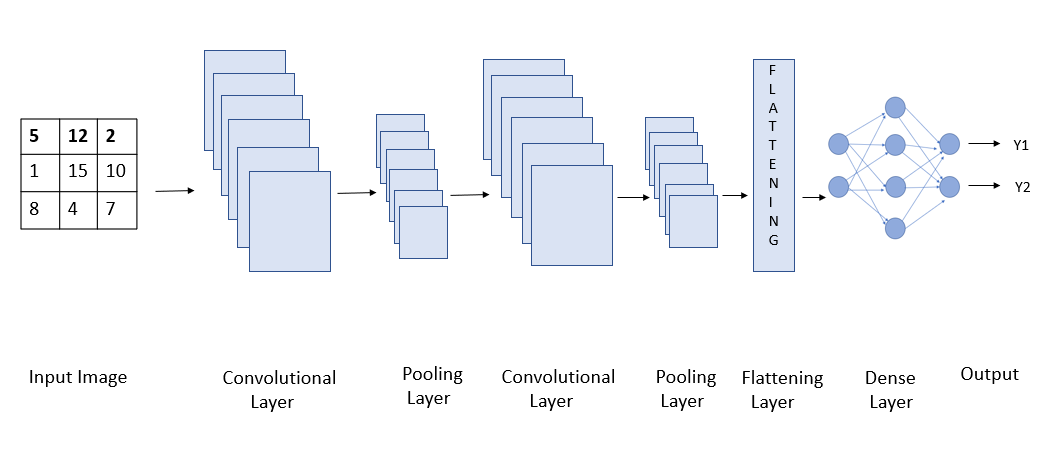# Deep Learning with CIFAR-10 Image ClassificationIn this article, we will be implementing a Deep Learning Model using CIFAR-10 dataset. The dataset is commonly used in Deep Learning for testing models of Image Classification.

Neural Networks are the programmable patterns that helps to solve complex problems and bring the best achievable output. Deep Learning as we all know is a step ahead of Machine Learning, and it helps to train the Neural Networks for getting the solution of questions unanswered and or improving the solution!

In this article, we will be implementing a Deep Learning Model using CIFAR-10 dataset. The dataset is commonly used in Deep Learning for testing models of Image Classification. It has 60,000 color images comprising of 10 different classes. The image size is 32x32 and the dataset has 50,000 training images and 10,000 test images. One can find the CIFAR-10 dataset here.

Importing Data

Deep Learning models require machine with high computational power. It is generally recommended to use online GPUs like that of Kaggle or Google Collaboratory for the same. I have implemented the project on Google Collaboratory. For the project we will be using TensorFlow and matplotlib library. Since the dataset is used globally, one can directly import the dataset from keras module of the TensorFlow library.

``````import tensorflow as tf
import matplotlib.pyplot as plt
from tensorflow.keras.datasets import cifar10 ``````

Pre-Processing the Data

The first step of any Machine Learning, Deep Learning or Data Science project is to pre-process the data. We will be defining the names of the classes, over which the dataset is distributed. There are 10 different classes of color images of size 32x32. Once we have set the class name. We need to normalize the image so that our model can train faster. The pixel range of a color image is 0–255. We will be dividing each pixel of the image by 255 so the pixel range will be between 0–1. Actually, we will be dividing it by 255.0 as it is a float operation. For the model, we will be using Convolutional Neural Networks (CNN).

``````## setting class names
class_names=[‘airplane’,’automobile’,’bird’,’cat’,’deer’,’dog’,’frog’,’horse’,’ship’,’truck’]

x_train=x_train/255.0
x_train.shape
x_test=x_test/255.0
x_test.shape``````

In the output of shape we see 4 values e.g. (50000,32,32,3). These 4 values are as follows: the first value, i.e.(50,000/10,000) shows the number of images. The second and third value shows the image size, i.e. image height and width. Here the image size is 32x32. The fourth value shows ‘3’, which shows RGB format, since the images we are using are color images.

## Artificial Intelligence, Machine Learning, Deep Learning

Artificial Intelligence (AI) will and is currently taking over an important role in our lives — not necessarily through intelligent robots.

## AI(Artificial Intelligence): The Business Benefits of Machine Learning

Enroll now at CETPA, the best Institute in India for Artificial Intelligence Online Training Course and Certification for students & working professionals & avail 50% instant discount.

## Deep Learning 101 —  Neural Networks Explained

The past few decades have witnessed a massive boom in the penetration as well as the power of computation, and amidst this information.

## Deep Learning Explained in Layman's Terms

Deep Learning Explained in Layman's Terms. In this post, you will get to learn deep learning through a simple explanation (layman terms) and examples.

## Transfer Learning in Image Classification

Experimental evaluation of how the size of the training dataset affects the performance of a classifier trained through Transfer Learning.